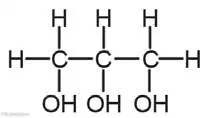# 4 Marks Question

1. Study the given structural formula and answer the following questions.1. Write the general formula of given structural formula.
2. The general formula of given structural formula is CnH2n+2
3. Write the name of the compound formed by the removal of one hydrogen by one 'OH' from the above compound.
4. The name of the compound formed by the removal of one hydrogen by one 'OH' from the above compound is Ethyl alcohol.
5. Write any one use of energy produced by the burning of the given compound.
6. To do welding in metals.
7. Write the structural formula of the compound which is formed by placing a double bond between two carbons in the given structural formula.
8. The structural formula of the compound which is formed by the placing double bond between two carbons in given structural formula is:2. Study the structural formula given below and answer the following questions:1. Write the IUPAC name of it.
2. Its IUPAC name is Propane-1, 2, 3 triol
3. In which group of organic compound is it kept?
4. It is kept in alcohol group.
5. Which type of hydrocarbon is formed if three 'OH' are replaced by three 'H' atom?
6. Propane is formed if three 'OH' are replaced by three 'H' atom.
7. Give two reasons to use this compound in fruits and food materials.
8. Two reasons to use this compound in fruits and food materials are:
1. To prevent fruits and foods from drying out.
2. It doesn't let fruits to rot.